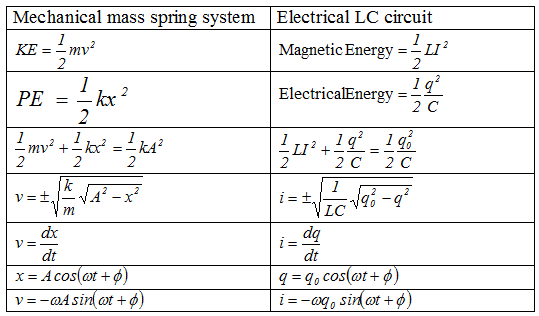# L-C circuit

## The L-C circuit

• We already know that capacitance and inductor can store electrical and magnetic energy respectively
• when a charged capacitor is allowed to discharge through an resistance less inductor ,the current oscillates back and forth in the circuit
• Thus electrical oscillations of constant amplitude are produced in the circuit and are called L-C oscillations
• Let the capacitor of capacitance C be given a charge q0 and is then connected to an inductor as shown below in the figure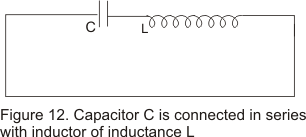• The moment the circuit is completed, charge on the capacitor begins to decrease giving rise to current in the circuit
• Suppose at any instant t during the discharge ,q is the amount of charge on the capacitor and I is the current through out the inductor
• EMF equation as obtained by Kirchhoff�s second law would be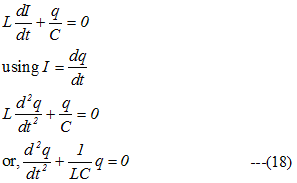• Comparing equation (18) with the SHM equation for mass spring system i.e.,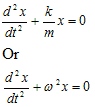where ω is the natural angular frequency of oscillations of undamped mass spring system, we can conclude that charge on the circuit oscillate with natural frequency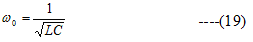and varies sinusoidal with time as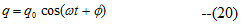where q0 is the maximum value of q and φ is the phase constant .
For initial phase φ=0,q=q0cosωt
• We thus see that LC circuit is identical to mass-spring system executing SHM
• Time period of oscillations is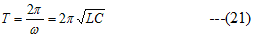and frequency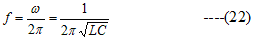• The current in the circuit is given by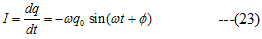Equation(23) indicates that the current in the circuit is also oscillatory and has the same frequency as charge
• In an L-C circuit ,during oscillations energy is partly electric and partly magnetic that is the oscillations consists of a transfer of energy back and forth from electric field of capacitor to magnetic field of the inductor
• The total energy of the circuit always remain constant and the situation is analogous to the transfer of energy in the mass-spring oscillation where energy alternates between two forms kinetic and potential
• Table given below compares the mechanic oscillations of mass spring system with that of electrical oscillations in an L-C circuit